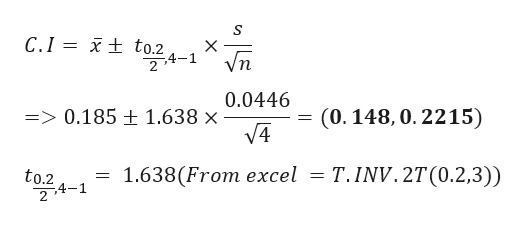# A physicist examines 4 seawater samples for iron concentration. The mean iron concentration for the sample data is 0.185 cc/cubic meter with a standard deviation of 0.0446. Determine the 80% confidence interval for the population mean iron concentration. Assume the population is approximately normal.Step 1 of 2 : Find the critical value that should be used in constructing the confidence interval. Round your answer to three decimal places.

Question
1 views

A physicist examines 4 seawater samples for iron concentration. The mean iron concentration for the sample data is 0.185 cc/cubic meter with a standard deviation of 0.0446. Determine the 80% confidence interval for the population mean iron concentration. Assume the population is approximately normal.

Step 1 of 2 :

Find the critical value that should be used in constructing the confidence interval. Round your answer to three decimal places.

check_circle

Step 1

Given data

Sample size(n) = 4

Sample mean = 0.185 cc/cubic

Sample standard deviation = 0.0446

Signi...help_outlineImage TranscriptioncloseC.I: x+ to.2 CO4-1" Vn 0.0446 (0. 148, 0. 2215) => 0.185 ± 1.638 × V4 T.INV. 2T(0.2,3)) 1.638(From eхсel to.2 2,4-1 fullscreen

### Want to see the full answer?

See Solution

#### Want to see this answer and more?

Solutions are written by subject experts who are available 24/7. Questions are typically answered within 1 hour.*

See Solution
*Response times may vary by subject and question.
Tagged in